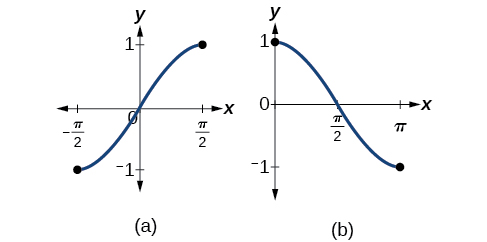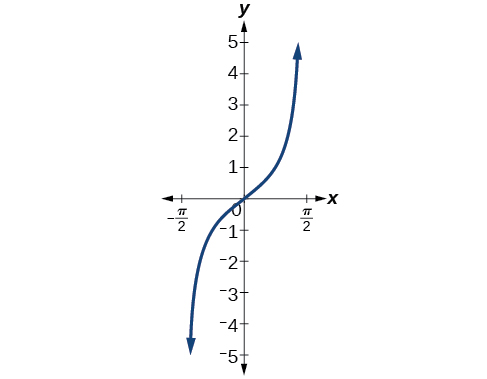# 8.3 Inverse trigonometric functions

 Page 1 / 15
In this section, you will:
• Understand and use the inverse sine, cosine, and tangent functions.
• Find the exact value of expressions involving the inverse sine, cosine, and tangent functions.
• Use a calculator to evaluate inverse trigonometric functions.
• Find exact values of composite functions with inverse trigonometric functions.

For any right triangle , given one other angle and the length of one side, we can figure out what the other angles and sides are. But what if we are given only two sides of a right triangle? We need a procedure that leads us from a ratio of sides to an angle. This is where the notion of an inverse to a trigonometric function comes into play. In this section, we will explore the inverse trigonometric functions .

## Understanding and using the inverse sine, cosine, and tangent functions

In order to use inverse trigonometric functions, we need to understand that an inverse trigonometric function “undoes” what the original trigonometric function “does,” as is the case with any other function and its inverse. In other words, the domain of the inverse function is the range of the original function, and vice versa, as summarized in [link] .

For example, if $\text{\hspace{0.17em}}f\left(x\right)=\mathrm{sin}\text{\hspace{0.17em}}x,\text{\hspace{0.17em}}$ then we would write $\text{\hspace{0.17em}}{f}^{-1}\left(x\right)={\mathrm{sin}}^{-1}x.\text{\hspace{0.17em}}$ Be aware that $\text{\hspace{0.17em}}{\mathrm{sin}}^{-1}x\text{\hspace{0.17em}}$ does not mean $\text{\hspace{0.17em}}\frac{1}{\mathrm{sin}x}.\text{\hspace{0.17em}}$ The following examples illustrate the inverse trigonometric functions:

• Since $\text{\hspace{0.17em}}\text{sin}\left(\frac{\pi }{6}\right)=\frac{1}{2},\text{\hspace{0.17em}}$ then $\text{\hspace{0.17em}}\frac{\pi }{6}={\text{sin}}^{-1}\left(\frac{1}{2}\right).$
• Since $\text{\hspace{0.17em}}\mathrm{cos}\left(\pi \right)=-1,\text{\hspace{0.17em}}$ then $\text{\hspace{0.17em}}\pi ={\mathrm{cos}}^{-1}\left(-1\right).$
• Since $\text{\hspace{0.17em}}\mathrm{tan}\left(\frac{\pi }{4}\right)=1,\text{\hspace{0.17em}}$ then $\text{\hspace{0.17em}}\frac{\pi }{4}={\mathrm{tan}}^{-1}\left(1\right).$

In previous sections, we evaluated the trigonometric functions at various angles, but at times we need to know what angle would yield a specific sine, cosine, or tangent value. For this, we need inverse functions. Recall that, for a one-to-one function    , if $\text{\hspace{0.17em}}f\left(a\right)=b,\text{\hspace{0.17em}}$ then an inverse function would satisfy $\text{\hspace{0.17em}}{f}^{-1}\left(b\right)=a.$

Bear in mind that the sine, cosine, and tangent functions are not one-to-one functions. The graph of each function would fail the horizontal line test. In fact, no periodic function can be one-to-one because each output in its range corresponds to at least one input in every period, and there are an infinite number of periods. As with other functions that are not one-to-one, we will need to restrict the domain    of each function to yield a new function that is one-to-one. We choose a domain for each function that includes the number 0. [link] shows the graph of the sine function limited to $\text{\hspace{0.17em}}\left[-\frac{\pi }{2},\frac{\pi }{2}\right]\text{\hspace{0.17em}}$ and the graph of the cosine function limited to $\text{\hspace{0.17em}}\left[0,\pi \right].$(a) Sine function on a restricted domain of   [ − π 2 , π 2 ] ;   (b) Cosine function on a restricted domain of   [ 0 , π ]

[link] shows the graph of the tangent function limited to $\text{\hspace{0.17em}}\left(-\frac{\pi }{2},\frac{\pi }{2}\right).$Tangent function on a restricted domain of   ( − π 2 , π 2 )

These conventional choices for the restricted domain are somewhat arbitrary, but they have important, helpful characteristics. Each domain includes the origin and some positive values, and most importantly, each results in a one-to-one function that is invertible. The conventional choice for the restricted domain of the tangent function also has the useful property that it extends from one vertical asymptote    to the next instead of being divided into two parts by an asymptote.

#### Questions & Answers

A laser rangefinder is locked on a comet approaching Earth. The distance g(x), in kilometers, of the comet after x days, for x in the interval 0 to 30 days, is given by g(x)=250,000csc(π30x). Graph g(x) on the interval [0, 35]. Evaluate g(5)  and interpret the information. What is the minimum distance between the comet and Earth? When does this occur? To which constant in the equation does this correspond? Find and discuss the meaning of any vertical asymptotes.
Kaitlyn Reply
The sequence is {1,-1,1-1.....} has
amit Reply
circular region of radious
Kainat Reply
how can we solve this problem
Joel Reply
Sin(A+B) = sinBcosA+cosBsinA
Eseka Reply
Prove it
Eseka
Please prove it
Eseka
hi
Joel
June needs 45 gallons of punch. 2 different coolers. Bigger cooler is 5 times as large as smaller cooler. How many gallons in each cooler?
Arleathia Reply
7.5 and 37.5
Nando
find the sum of 28th term of the AP 3+10+17+---------
Prince Reply
I think you should say "28 terms" instead of "28th term"
Vedant
the 28th term is 175
Nando
192
Kenneth
if sequence sn is a such that sn>0 for all n and lim sn=0than prove that lim (s1 s2............ sn) ke hole power n =n
SANDESH Reply
write down the polynomial function with root 1/3,2,-3 with solution
Gift Reply
if A and B are subspaces of V prove that (A+B)/B=A/(A-B)
Pream Reply
write down the value of each of the following in surd form a)cos(-65°) b)sin(-180°)c)tan(225°)d)tan(135°)
Oroke Reply
Prove that (sinA/1-cosA - 1-cosA/sinA) (cosA/1-sinA - 1-sinA/cosA) = 4
kiruba Reply
what is the answer to dividing negative index
Morosi Reply
In a triangle ABC prove that. (b+c)cosA+(c+a)cosB+(a+b)cisC=a+b+c.
Shivam Reply
give me the waec 2019 questions
Aaron Reply

### Read also:

#### Get the best Algebra and trigonometry course in your pocket!

Source:  OpenStax, Algebra and trigonometry. OpenStax CNX. Nov 14, 2016 Download for free at https://legacy.cnx.org/content/col11758/1.6
Google Play and the Google Play logo are trademarks of Google Inc.

Notification Switch

Would you like to follow the 'Algebra and trigonometry' conversation and receive update notifications?ByByByBy